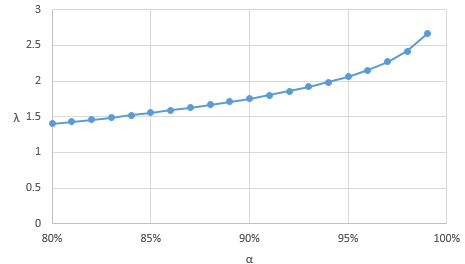# Expected Shortfall closed-form for Normal distribution

Today, I would like to share a little exercise I did to compute the Expected Shortfall of a normal variable. For those of you who are not familiar with this risk measure, it evaluates the average of the $(1-\alpha)$-worst outcomes of a probability distribution (example and formal definition follow).

If you have enough data, the expected shortfall can be empirically estimated. Let’s say we want to compute the expected shortfall at 95% (denoted $ES_\text{95%}$) and that we have 1000 points. The first thing to do is to sort the 1000 points. Then, we take the $1-95\%=5\%$ worst outcomes, which is for 1000 points $1000 \cdot 5\% = 50$ points. Finally, we simply average these points to get our estimated expected shortfall. Nevertheless, this is only an estimate and it will be precise only if you have enough points available.

Sometimes, you can assume the distribution of the points and when these points are a set of returns, it is quite common to assume that they follow some normal distribution $\mathcal{N}(\mu,\sigma^2)$. In this case, you can find a closed form formula which gives you the exact solution for a given $\alpha$, given the parameters $\mu$ and $\sigma$. This result is available in several papers but usually comes without the proof, which I will provide below.

Let’s assume we have a random variable $X \sim \mathcal{N}(\mu,\sigma^2)$. The first thing to do is to re-express this variable in term of a standard normal variable $Z \sim \mathcal{N}(0,1)$. This is easily done as follows:

$$X = \mu + \sigma Z$$

The next thing to do is to express the Value at Risk at a certain level $\alpha$, which is defined as follows:

$$\text{VaR}_\alpha (X) = \{ Y ~ | ~ \mathbb{P}(X \leq Y) = 1-\alpha \}$$

This can be easily found as well, since:
\begin{align} \mathbb{P}(X \leq Y) &= \mathbb{P}( \mu + \sigma Z \leq Y)\\ &= \mathbb{P} \left(Z \leq \frac{Y-\mu}{\sigma} \right)\\ &= \Phi \left( \frac{Y-\mu}{\sigma} \right) = 1-\alpha\\ \end{align}
where $\Phi(\cdot)$ is the cumulative standard normal distribution.

We can then deduce that:
$$\text{VaR}_\alpha (X) = Y = \Phi^{-1}(1-\alpha) \sigma + \mu$$
where $\Phi^{-1}(\cdot)$ is the inverse cumulative standard normal distribution and which can be looked up online.

Now, we can actually start working on the closed-form. Let’s first express the expected shortfall in terms of the value at risk:
$$ES_\alpha(X) = \frac{1}{1-\alpha} \int_\alpha^1 \text{VaR}_u(X) du$$

Using our previous result, we can rewrite the definition above as follows:
\begin{align} ES_\alpha(X) &= \frac{1}{1-\alpha} \int_\alpha^1 ( \Phi^{-1}(1-u) \sigma + \mu ) du\\ &= \frac{1}{1-\alpha} \left( \int_\alpha^1 \Phi^{-1}(1-u) \sigma du + \int_\alpha^1 \mu du \right)\\ &= \frac{1}{1-\alpha} \int_\alpha^1 \Phi^{-1}(1-u) \sigma du + \mu \end{align}

As you can see, we managed to split the big integral into two parts, one of which is solved very easily. However, there remains an integral with $\Phi^{-1}(\cdot)$ which is a very complex function so we need to find a way around. To do this, we are going to use a change of variable introducing $u = \Phi(y)$. We have then $y = \Phi^{-1}(u)$ and $du = \phi(y)dy$ where $\phi(y)=\frac{1}{\sqrt{2\pi}} \exp \left( – \frac{y^2}{2} \right)$ is the standard normal density function.

\begin{align} \int_\alpha^1 \Phi^{-1}(1-u) \sigma du &= \int_{\Phi^{-1} (\alpha)}^{\Phi^{-1}(1)} \Phi^{-1}\left(\underbrace{ 1-\Phi(y)}_{\Phi(-y)} \right) \sigma \phi (y) dy \\ &= \int_{\Phi^{-1} (\alpha)}^\infty -y \sigma \phi (y) dy \\ &= \int_{\Phi^{-1} (\alpha)}^\infty -\frac{y \sigma}{\sqrt{2\pi}} \exp \left( – \frac{y^2}{2} \right) dy \\ \end{align}

So, we got rid of $\Phi^{-1}(\cdot)$ and the remaining integral is relatively easy to solve:

\begin{align} \int_{\Phi^{-1} (\alpha)}^\infty -\frac{y \sigma}{\sqrt{2\pi}} \exp \left( – \frac{y^2}{2} \right) dy &= \frac{\sigma}{\sqrt{2\pi}} \int_{\Phi^{-1} (\alpha)}^\infty -y \exp \left( – \frac{y^2}{2} \right) dy\\ &= \frac{\sigma}{\sqrt{2\pi}} \left( \exp \left( – \frac{y^2}{2} \right)\right)|_{\Phi^{-1} (\alpha)}^\infty\\ &= \frac{\sigma}{\sqrt{2\pi}} \left( 0 – \exp \left( – \frac{\Phi^{-1} (\alpha)^2}{2} \right)\right)\\ &= -\sigma \frac{1}{\sqrt{2\pi}} \exp \left( – \frac{\Phi^{-1} (\alpha)^2}{2} \right)\\ &= -\sigma \phi \left( \Phi^{-1} (\alpha) \right)\\ \end{align}

Now, we just have to include this in our previous result to get:

\begin{align} ES_\alpha(X) &= \frac{1}{1-\alpha} \int_\alpha^1 \Phi^{-1}(1-u) \sigma du + \mu\\ &= \frac{1}{1-\alpha} \left[ -\sigma \phi \left( \Phi^{-1} (\alpha) \right) \right] + \mu\\ &= \mu – \sigma \cdot \frac{\phi \left( \Phi^{-1} (\alpha) \right)}{1-\alpha} \end{align}

This is a very good result, since we know that $\lambda( \alpha ) = \frac{\phi \left( \Phi^{-1} (\alpha) \right)}{1-\alpha}$ is a function of $\alpha$ that can be computed very easily, for example in Excel. Below are the results for $\alpha$ from 80% to 99%.Values of $\lambda$ for different $\alpha$

That’s it, with this simple formula, you can compute the expected shortfall of a normally distributed variable without needing any data point.